Courses

# Cables (Part - 2) Civil Engineering (CE) Notes | EduRev

## Civil Engineering (CE) : Cables (Part - 2) Civil Engineering (CE) Notes | EduRev

The document Cables (Part - 2) Civil Engineering (CE) Notes | EduRev is a part of the Civil Engineering (CE) Course Structural Analysis.
All you need of Civil Engineering (CE) at this link: Civil Engineering (CE)

Example 31.1
Determine reaction components at A and B, tension in the cable and the sag yB , and yE of the cable shown in Fig. 31.4a. Neglect the self weight of the cable in the analysis.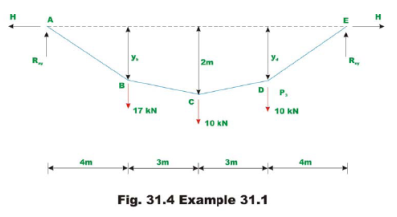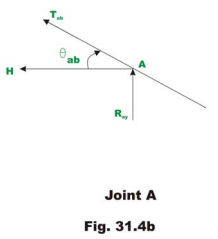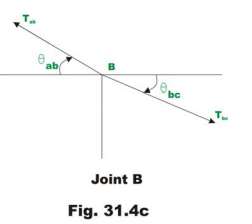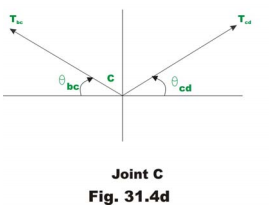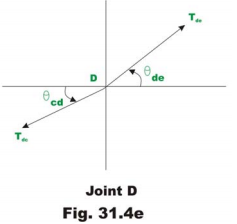Since there are no horizontal loads, horizontal reactions at A and B should be the same. Taking moment about E, yields

Ray ×14 - 17×20− 10×7 − 10×4 = 0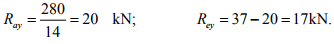Now horizontal reaction H may be evaluated taking moment about point C of all forces left of C.

Ray ×7−H×2 -17×3 = 0

H = 44.5 kN

Taking moment about B of all the forces left of B and setting MB = 0, we get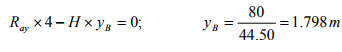Similarly,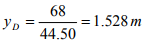To determine the tension in the cable in the segment AB , consider the equilibrium of joint A (vide Fig.31.4b).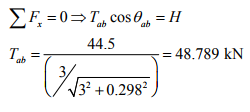The tension Tab may also be obtained as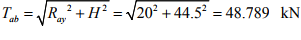Now considering equilibrium of joint BC and D one could calculate tension in different segments of the cable.

Segment bc
Applying equations of equilibrium,

∑ Fx = 0 ⇒ Tab cosθab =Tbc cosθbc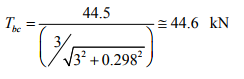See Fig.31.4c

Segment cd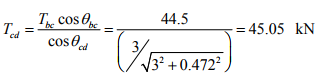See Fig.31.4d.
See Fig.31.4e.

Segment de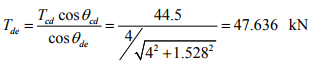The tension Tdc may also be obtained as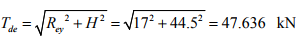Example 31.2
A cable of uniform cross section is used to span a distance of 40m as shown in Fig 31.5. The cable is subjected to uniformly distributed load of 10 kN/m. run. The left support is below the right support by 2 m and the lowest point on the cable C is located below left support by 1 m. Evaluate the reactions and the maximum and minimum values of tension in the cable.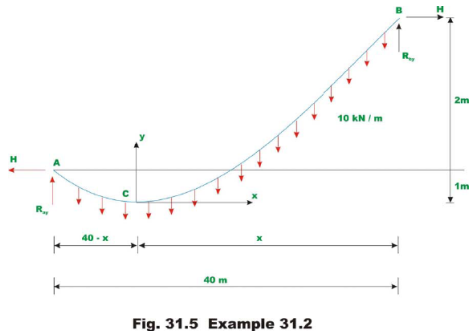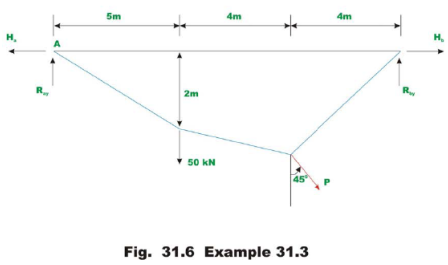Assume the lowest point C to be at distance of x m from B . Let us place our origin of the co-ordinate system xy at C . Using equation 31.5, one could write,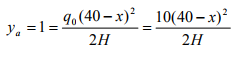(1)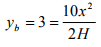(2)

where ya and yb be the y co-ordinates of supports A and B respectively. From equations 1 and 2, one could evaluate the value of x . Thus,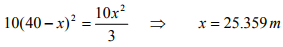From equation 2, the horizontal reaction can be determined.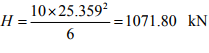Now taking moment about A of all the forces acting on the cable, yields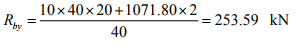Writing equation of moment equilibrium at point B , yields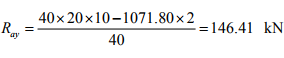Tension in the cable at supports A and B are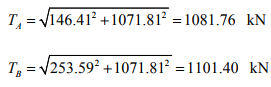The tension in the cable is maximum where the slope is maximum as T cosθ = H. The maximum cable tension occurs at B and the minimum cable tension occurs at C where dy/dx = θ = 0 and TC = H =1071.81 kN

Example 31.3
A cable of uniform cross section is used to support the loading shown in Fig 31.6. Determine the reactions at two supports and the unknown sag yC.

Taking moment of all the forces about support B ,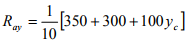(1)

Ray = 65+ 10yc

Taking moment about B of all the forces left of B and setting MB = 0, we get,

Ray × 3 - Ha × 2 = 0

⇒ Ha = 1.5 Ray                           (2)

Taking moment about C of all the forces left of C and setting MC = 0 , we get

∑MC = 0     Ray × 7 - Ha × yC - 50 × 4 = 0

Substituting the value of Ha in terms of Ray in the above equation,

7 Ray - 1.5 Ray yC - 200 = 0                          (3)

Using equation (1), the above equation may be written as,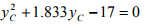(4)

Solving the above quadratic equation, yC can be evaluated. Hence,

yC = 3.307m.

Substituting the value of yC in equation (1),

Ray = 98.07 kN

From equation (2),

Ha = 1.5Ray = 147.05 kN

Now the vertical reaction at D, Rdy is calculated by taking moment of all the forces about A ,

Rdy × 10 -100 × 7 +100 × 3.307 - 50 × 3 = 0

Rdy = 51.93 kN.

Taking moment of all the forces right of C about C, and noting that ∑ MC = 0,

Rdy × 3 = Hd × yc   ⇒ Hd = 47.109 kN.

Summary

In this lesson, the cable is defined as the structure in pure tension having the funicular shape of the load. The procedures to analyse cables carrying concentrated load and uniformly distributed loads are developed. A few numerical examples are solved to show the application of these methods to actual problems.

Offer running on EduRev: Apply code STAYHOME200 to get INR 200 off on our premium plan EduRev Infinity!

## Structural Analysis

30 videos|122 docs|28 tests

,

,

,

,

,

,

,

,

,

,

,

,

,

,

,

,

,

,

,

,

,

;